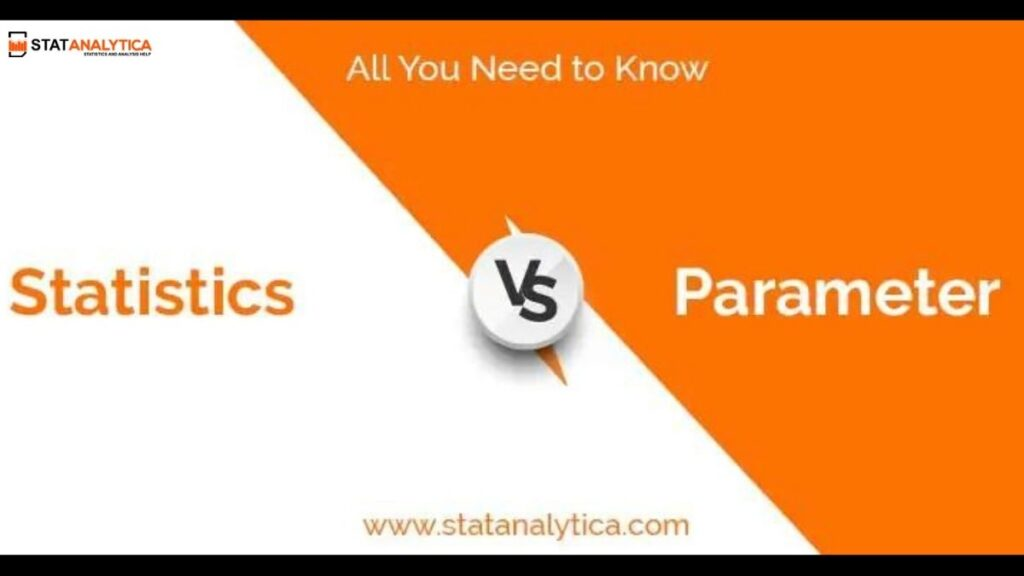# Statistics Vs Parameter: All You Need to Know

When I was a child, I always thought, how do news reporters know that the storm is coming? And how do people say that a particular hospital has admitted the maximum number of accident patients? How is all this possible?

But when I grew up, I learned about statistics and parameters. Now, I know statistics used for weather forecasting and parameter concepts used to know the maximum number of accidents registered in a particular hospital.

But several people have doubts about the statistics vs parameter. Therefore, it becomes necessary to understand the basic difference between these two terms.

But several students face difficulty in understanding the terms statistics and parameter.

Both terms might look similar, but there is a difference between these two. Statistics and parameter are the two terms used to determine the value of a given sample size.

A parameter considers each person who belongs to the entire group. At the same time, statistics involve the data that it gets from the given samples by ignoring the rest of the community’s appearance. If you still find any difficulty in understanding these two terms, then continue reading this post.

## What are the parameters?

Before proceeding to the statistics vs parameter, let’s get some information about the parameters and the statistics?

A parameter represents the characteristics of the whole population. The characteristics may be the median, mean, or mode of the data. Those are derived from the components which are taken as a whole.

Here, the population term can include each unit that consists of a familiar character. And is relevant to the attributes of the study.

## What are the statistics?

Just like a parameter, statistics is used to consider a sample of the whole population. It might be a random sample or an outcome of a few predefined parameters.

We use them to select the sample. Whereas in statistics, there is no need to consider each unit of the population. But the size of the given sample must be large enough that it can ensure the accuracy of the obtained information.

Despite less accuracy, statistics are used when you need to gather the data from a large range of populations whose single unit is not precise to be accountable for.

To get better statistics’ accuracy, you need to rely on previous data and analytical tools like standard deviation and variance.

### Example of statistics

We will now discuss the major difference between statistics versus parameter in the tabular form described below.

## Symbol notation of statistics vs parameter

In parameter: Population proportion is described by P, mean is described by µ (Greek letter mu). σ2 shows variance. N shows population size, σ (Greek letter sigma) shows standard deviation, σx̄ shows Standard error of mean, σ/µ shows Coefficient of variation, (X-µ)/σ shows standardized variate (z), and σp shows standard error of population.

In statistics: Mean is described by (x-bar), sample proportion is described by (p-hat). s shows standard deviation, s2 shows variance. The sample size is described by n, sx̄ shows Standard error of the mean. sp shows the standard error of a proportion, s/(x̄) shows the Coefficient of variation, and (x-x̄)/s outlines standardized variate (z).

## Statistics vs Parameter (tabular form)

This table has all the key differences between statistics vs parameter that helps you to understand the basic differences between them.

## Quiz: Statistics vs Parameter

Let’s check what you have learned from the above paragraphs! Select the correct answer that is whether the statement represents the statistics or parameter concept.

1. More than 2 from 25 teens have been diagnosed with depression or anxiety.

(A) Statistics

(B) Parameter

1. Latvian women consider being the tallest on the earth, which has a mean height of 170cm.

(A) Statistics

(B) Parameter

1. The average final maths exam of a high school has increased from 69% to 77% in the last decades.

(A) Statistics

(B) Parameter

1. The average annual income of 40 employees is \$44,000 at company X.

(A) Statistics

(B) Parameter

## So, what should I prefer, statistics or parameter?

If a data scientist assigns to get more accurate results to the output data, it would be helpful to go with the statistics. The large the data of the population, the accurate the answer.

At the same time, the parameter is used to define the particular population. The less the data is to measure, the less accuracy of the experiment. It disables the users to get the mean value of the overall sample.

So, I would recommend you to go with the statistics if you have the large data to carry out the accurate results. But in case you need a particular solution from a particular group of people of a survey, then go with the parameter.

## Conclusion

This blog has provided all the necessary information about statistics vs parameter. As it provides the definition of parameters and statistics homework help with its examples. Besides this, this post has a table that differentiates both of the terms; it also clarifies that both terms might seem similar but has the differences in between them. Therefore, this table helps you know those differences and remember all the notations that you use while solving the statistics problems.

The use of statistics and parameter varies from purpose to purpose. It means statistics can apply to the different problem and parameter is applied to the different problem. That is why it becomes necessary that you know where to implement the concept of statistics and implement the parameter concept.

I have detailed all the necessary differences between statistics vs parameter. Moreover, I have suggested to the readers which concept they should go with and when to use it.

Even though you find any difficulty in statistics or parameter assignments, you can use our services as we provide high-quality data with plagiarism-free reports.